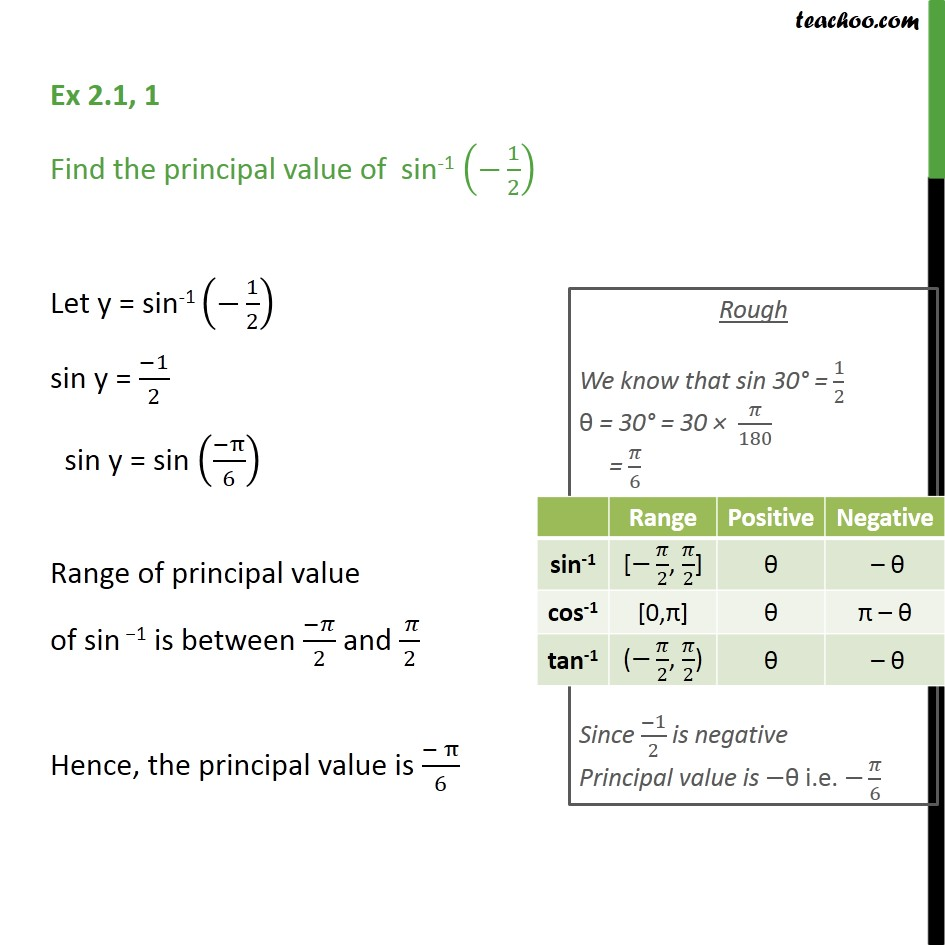1. Chapter 2 Class 12 Inverse Trigonometric Functions
2. Serial order wise
3. Ex 2.1

Transcript

Ex 2.1, 1 Find the principal value of sin-1 (−1/2) Let y = sin-1 (−1/2) sin y = (−1)/2 sin y = sin ((−π)/6) Range of principal value of sin −1 is between (−𝜋)/2 and ( 𝜋)/2 Hence, the principal value is (− π)/6

Ex 2.1